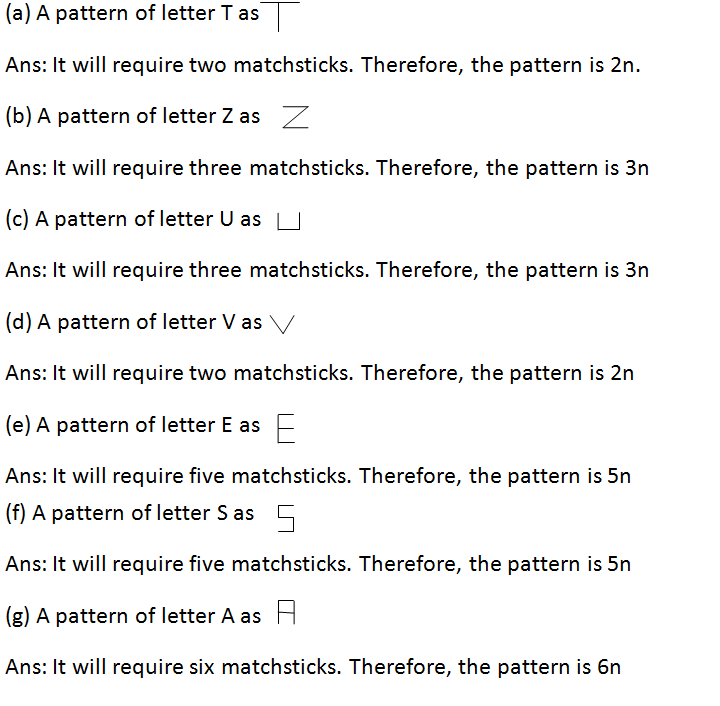# NCERT Class 6 Mathematics Eleventh Chapter Algebra Exercise 11.1 Solution

## EXERCISE 11.1

(1) Find the rule which gives the number of matchsticks required to make the following matchstick patterns. Use a variable to write the rule.(2) We already know the rule for the pattern of letters L, C and F. Some of the letters from Q.1 (given above) give us the same rule as that given by L. Which are these? Why does this happen?

Solution: (a) and (d); The number of matchsticks required in each of them is 2.

(3) Cadets are marching in a parade. There are 5 cadets in a row. What is the rule which gives the number of cadets, given the number of rows? (Use n for the number of rows.)

Solution: Lets the number of rows is n.

There are 5 cadets in a row.

Therefore, the total number of cadets = 5 × n = 5n

(4) If there are 50 mangoes in a box, how will you write the total number of mangoes in terms of the number of boxes? (Use b for the number of boxes.)

Solution: Lets the number of boxes is b.

There are 50 mangoes in a box.

So, the total number of boxes = 50 × b = 50b

(5) The teacher distributes 5 pencils per student. Can you tell how many pencils are needed, given the number of students? (Use s for the number of students.)

Solution: Lets the number of students is s.

The teacher distributes 5 pencils per student.

Therefore, the number of pencils which is needed = 5 × s = 5s

In case you are missed :- NCERT Solution for Whole Numbers Exercise 2.3

(6) A bird flies 1 kilometer in one minute. Can you express the distance covered by the bird in terms of its flying time in minutes? (Use t for flying time in minutes.)

Solution: Lets the time of birds is flying t minutes.

In one minute the bird covered 1 km.

Therefore, the covered distance = 1 × t = t km.

(7) Radha is drawing a dot Rangoli (a beautiful pattern of lines joining dots) with chalk powder. She has 9 dots in a row. How many dots will her Rangoli have for r rows? How many dots are there if there are 8 rows? If there are 10 rows?

Solution: There the number of dots in a row = 9

Therefore, the number of dots in r rows = 9 × r = 9r

If there are 8 rows, so the number of dots = 9 × 8 = 72 dots

If there are 10 rows, that the number of dots = 9 × 10 = 90 dots.

(8) Leela is Radha’s younger sister. Leela is 4 years younger than Radha. Can you write Leela’s age in terms of Radha’s age? Take Radha’s age to be x years.

Solution: let the age of radha is x years.

Leela is 4 years younger than radha.

Therefore, Leela’s age is = (x – 4) years.

(9) Mother has made laddus. She gives some laddus to guests and family members; still 5 laddus remain. If the number of laddus mother gave away is l, how many laddus did she make?

Solution: Number of laddus that mother gave away is l.

Number of laddus remain after gave to guests is 5.

Therefore, she make the total number of laddus = (l + 5 )

(10) Oranges are to be transferred from larger boxes into smaller boxes. When a large box is emptied, the oranges from it fill two smaller boxes and still 10 oranges remain outside. If the number of oranges in a small box are taken to be x, what is the number of oranges in the larger box?

Solution: The number of oranges in a small box are x.

The oranges in two small boxes are 2x.

The number of oranges left = 10.

Therefore, the number of oranges in the larger box = (2x + 10)

(11) (a) Look at the following matchstick pattern of squares(Fig 11.6). The squares are not separate. Two neighbouring squares have a common matchstick. Observe the patterns and find the rule that gives the number of matchsticks in terms of the number of squares. (Hint: If you remove the vertical stick at the end, you will get a pattern of Cs.)Solution: The number of matchsticks is 4, 7, 10, 13, which is one more thrice of the number of squares in the pattern.

Therefore, the pattern is = 3n + 1.

(b) Fig 11.7 gives a matchstick pattern of triangles. As in Exercise 11 (a) above, find the general rule that gives the number of matchsticks in terms of the number of triangles.Solution: The number of matchsticks is 3, 5, 7, 9, which is 1 more than twice of the number of triangle in the pattern.

Therefore, the pattern is = 2n + 1.

In case you are missed :- Next Chapter Exercise Solution

Updated: April 14, 2023 — 3:20 pm